﻿Relative Strength Index

# Relative Strength Index

The Relative Strength Index (Rsi) calculates the ratio of the recent upward price movements to the absolute price movement.  The Rsi ranges from 0 to 100.  The Rsi is interpreted as an overbought/oversold indicator when the value is over 70/below 30.  You can also look for divergence with price. If the price is making new highs/lows, and the Rsi is not, it indicates a reversal.Market Signals

A popular method of analyzing the Rsi is to look for a divergence in which the security is making a new high, but the Rsi is failing to surpass its previous high.  This divergence foreshadows an impending reversal.  When the Relative Strength Index then turns down and falls below its most recent trough, it is said to have completed a "failure swing".  The failure swing is considered a confirmation of the expected reversal.Calculation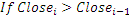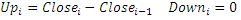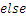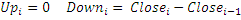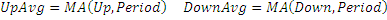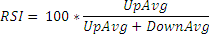Chart Example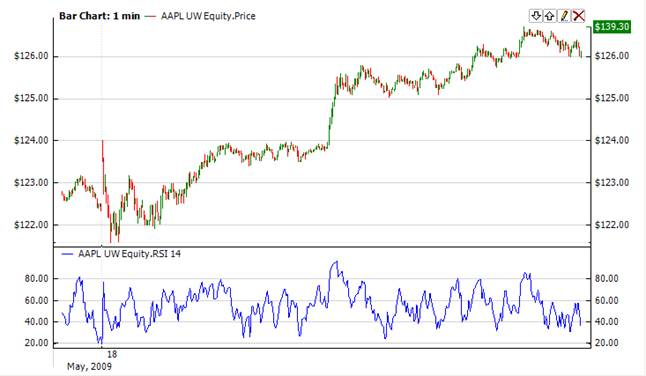Implementation and Usage

To initialize Rsi use one of the following constructors:

Rsi – sets default values: period = 14

Rsi(Int32, Boolean) – sets value for period

Rsi(IAverager, IAverager, Boolean) – sets the type of averaging for UpAvg and DownAvg (see formula)

Use

RSI - property to get current valueExample
C#
``` 1// Create new instance
2Rsi rsi = new Rsi(28);
3
4// Number of stored values
5rsi.HistoryCapacity = 2;
6